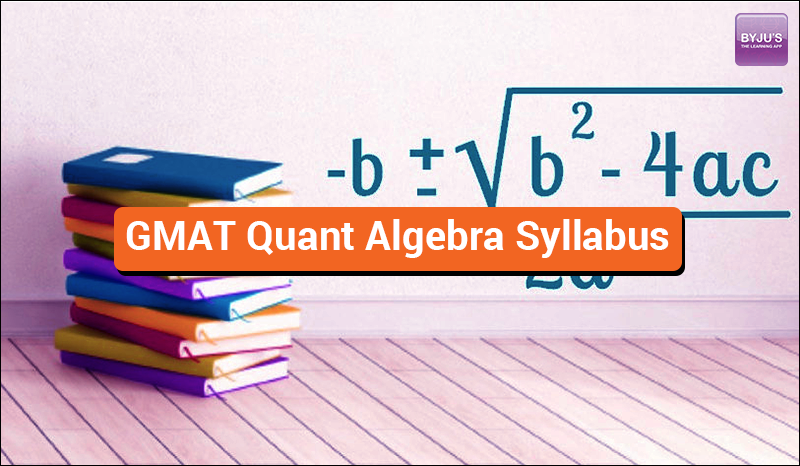# GMAT Quant Algebra SyllabusGMAT Algebra Syllabus

Sums on algebra for GMAT exam consists of the following primary topics –

1. Absolute Value
2. Exponential Equations
3. Exponential Powers
5. Inequalities
7. Multiplying & Dividing
8. Absolute Value
9. Exponents
10. Linear Equations
11. Order of Operations
13. Factoring
14. Simplifying Equations
15. Simultaneous Equations

Absolute Value

Definition, Solving Equations & Working With Multiple Absolute Values

Exponential Equations

1. Basic Concepts – Base, Exponent, Radical, Exponential Expression, Exponential Equation
2. Laws of Exponents

$a^{0} = 1$ $0^{n} = 0 ; n>0$ $a^{1} = a$ $(-1)^{n} = \left\{\begin{matrix} 1 & , n \; even\\ -1 & n \; odd \end{matrix}\right.$ $a^{n} . a^{m} = a^{n+m}$ $a^{n} . b^{n} = (a.b)^n$ $\frac{a^{n}}{a^{m}} = a^{n-m}$ $\frac{a^{n}}{b^{n}} = \left ( \frac{a}{b} \right )^{n}$ $(b^{n})^{m} = b^{(n^{m})}$ $\sqrt[m]{b^{n}} = b^{\frac{n}{m}}$ $b^{\frac{1}{n}} = \sqrt[n]{b}$ $a^{-n} = \frac{1}{a^{n}}$

1. Exponential Powers – Rules of Exponents
$\frac{x^{n}}{x^{m}} = x^{n-m}$ $x^{n}x^{m} = x^{n+m}$ $x^{n}y^{n} = (xy)^{n}$ $\left ( \frac{x}{y} \right )^{n} = \frac{x^{n}}{y^{n}}$ $x^{-n} = \frac{1}{x^{n}}$ $(x^{y})^{z} = x^{y.z}$

$a^{\frac{x}{y}} = \sqrt[y]{a^{x}}$ $\sqrt{x}.\sqrt{n} = \sqrt{xn}$ $\sqrt{\frac{x}{y}} = \frac{\sqrt{x}}{\sqrt{y}}$ $(\sqrt{x})^{n} = \sqrt{x^{n}}$ $a\sqrt{c} + b\sqrt{c} = (a+b)\sqrt{c})$ $\sqrt{a} + \sqrt{b} \neq \sqrt{a+b}$

**NOTE – It’s recommended for the aspirants to memorize the types of Radicals –

$\sqrt{2} \approx 1.41 \; and \; (1.41)^{2} \approx 2$ $\sqrt{3} \approx 1.73 \; and \; (1.73)^{2} \approx 3$ $\sqrt{11} = 11 \; and \; (11)^{2} = 121$ $\sqrt{169} = 13 \; and \; (13)^{2} = 169$ $\sqrt{225} = 15 \; and \; (15)^{2} = 225$ $\sqrt{625} = 25 \; and \; (25)^{2} = 625$

1. Inequalities

Types of Inequalities – Greater Than (>), Less Than (<), Greater Than or Equal to (>), Less Than or Equal to (<) and Not Equal to (≠). Adding & Subtracting, Multiplying & Dividing, Absolute Value, Exponents.

1. Linear Equations

Example –

$5x + 7y = 14$

Non-Linear Equations

Example –

$x^{2} -2x + 1 = 0$ $x^{-3} + y^{-3} + 9x^{2} + 2= 0$

1. Order of Operations

To solve a fundamental mathematical operation, the order of operations must be followed, which is –

P – Parentheses
E – Exponents
M – Multiplication
D – Division
S – Subtraction

$x=\frac{-b\pm \sqrt{b^{2}-4ac}}{2a}$
$a^{2} – b^{2} = (a+b)(a-b)$ $a^{2} + 2ab + b^{2} = (a+b)^{2}$ $a^{2} – 2ab + b^{2} = (a-b)^{2}$